# Python Object Oriented Programming: Complete Guide

1
58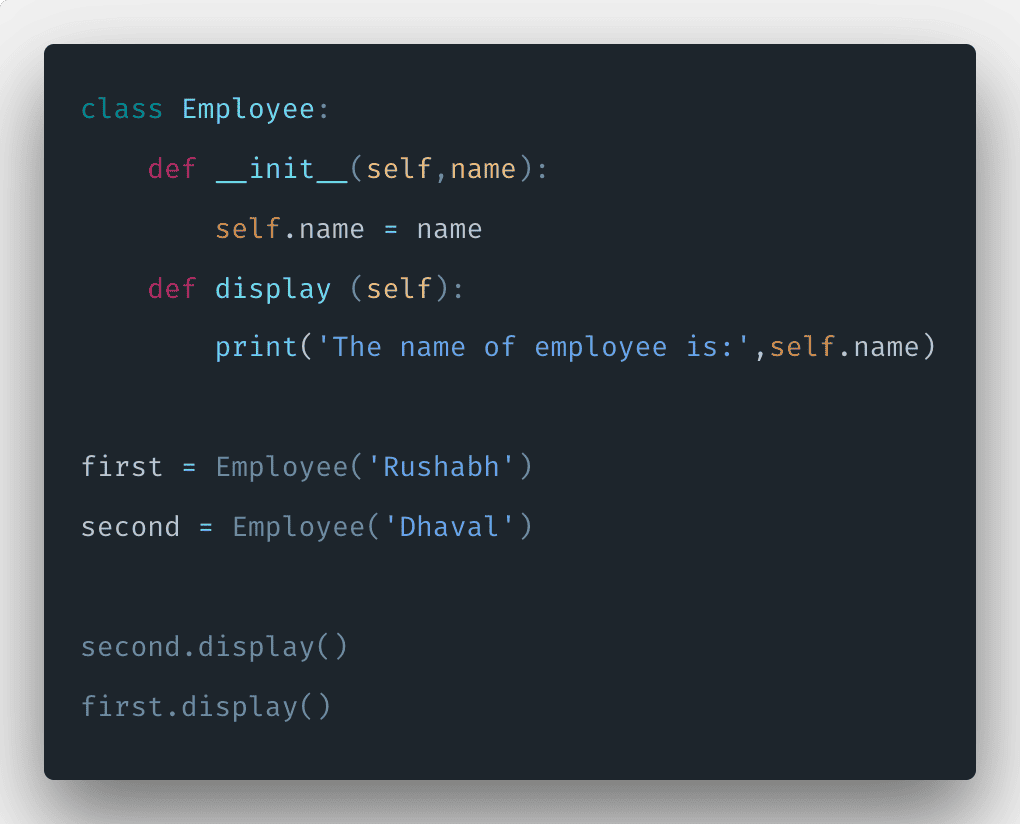Python Object oriented Programming, or OOP for short is a programming approach that provides the means of structuring the programs so that properties and behaviors are bundled into individual objects.

In this article, you will learn about Object-Oriented Programming in Python and their fundamental concepts with examples.

Object oriented programming in Python is an approach to modeling concrete, real-world things like cars as well as the relationship between things like companies and employees, students and teachers, etc.  In this post, you will learn the following concepts in the OOP paradigm.

1. Classes in Python
2. Objects in Python
3. Instance Methods
4. Instance Attributes
5. Init Constructor
6. Encapsulation
7. Inheritance

## Python Object-Oriented Programming

Objects are the center of the object-oriented programming paradigm,  which is not only representing the actual data, as in procedural programming, but in the overall structure of the program as well. Python is the multi-paradigm programming language. Meaning, it supports different programming approaches. The best approach to programming, in my opinion, is OOP.

## Class in Python

Classes in Python are used to create new user-defined(UDF) data structures that contain arbitrary information about something. It’s important to note that a class provides structure because it is a blueprint for how something should be defined, but it doesn’t offer any real content itself. For that, we need to create an object of that class.

We can think of the class as an entity with labels. It contains all the details about the name, size, color, etc. Based on these details, we can study human. Here, the human is an object.

Classes are like factories, and instances are constructed from the class, which has all the attributes and properties of the class. Classes are instance factories and instance factories.

### Define a class in Python

Let us define an empty class.

```# app.py

class FirstClass():
pass```

Here, we use the class keyword to define an empty class FirstClass. Also, we used Python’s reserved keyword pass here. This is very often used as a place holder where the code will eventually go. It allows us to run the above code without giving an error.

Define a property in a class.

```# app.py

class FirstClass():
age = 25```

Here, we have defined one property in the class called age.

We can also define a method inside the class.

```# app.py

class FirstClass():
def getColor(self):
return 'red'```

Here, function getColor() has always one argument called self, which is the object itself. So when we create an object of the above class, it will pass an object as a first argument.

## Objects in Python

Objects are the instances created from the class.  An instance is a specific object created from a particular class. You can create as many objects as you want from one class. It has classes’ methods and properties. While the class is a blueprint, an instance is a copy of the class with actual values.

Variables, methods are all defined in a class that is accessible to its objects. Let us create an instance of the MyFirst() class.

```# app.py

class FirstClass():
def getColor(self):
return 'red'

member = FirstClass()
print(member)```

In the above code, we have created an instance called a member, and we can see the information of that object by printing in the log.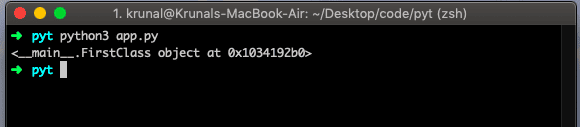We can create as many instances as you want.

```# app.py

class FirstClass():
def getColor(self):
return 'red'

instance = FirstClass()
instanceSecond = FirstClass()
instanceThird = FirstClass()

print(instance)
print(instanceSecond)
print(instanceThird)```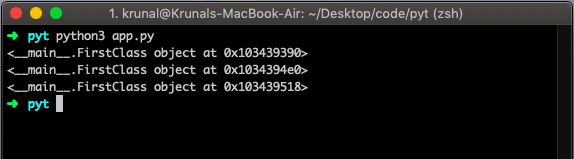## Instance Methods

The syntax of an instance method is somewhat different than another programming language. In another programming language, if the method defined in the class that has parameters or arguments then, you must provide an argument when it is called; otherwise, you will get an error.

In Python, when you define any method in a class, you will have to provide an argument called self.

It is by default argument to any instances methods. What it means that when you create an object from that class, that object itself will pass in that method.

You do not need to provide any argument at the time of calling that function, but the argument is a must when you define that function inside that class.

Let us take an example of that.

```# app.py

class FirstClass():
def getColor(self):
return 'red'

instance = FirstClass()

print(instance.getColor())
```

In the above example, we have defined a function inside the class called getColor() and pass one argument called self.

Now, the next step is to create an instance of that class and call that method but wait; we have not provided any argument when we have called that function.

See, this is the behavior of the method in Python. When you call any method on an instance, the first argument itself is an instance of a class. Now, run the above file and see the output.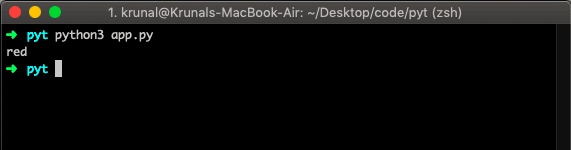Now, if you do not write any argument in method while defining any method in a class, then you will get an error. See the following example.

```# app.py

class FirstClass():
def getColor():
return 'red'

instance = FirstClass()

print(instance.getColor())
```

My editor Visual Studio Code also shows me an error.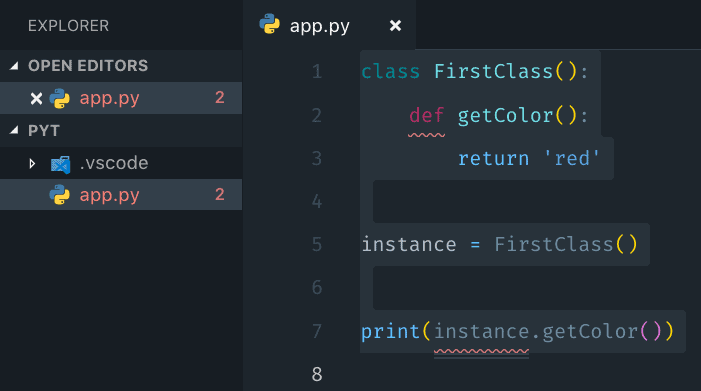After running the file, you will see an error in the console like below.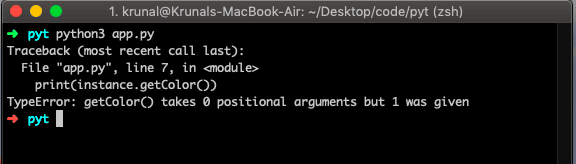## Instance Attributes

An object is a unit of data that has more than one attribute of a particular class or type. An instance can access the variables defined in the class. An instance can set and get the values in itself.

An instance data takes the form of instance attribute values, set and accessed through object.attribute syntax.

Let see an example below.

```# app.py

import random

class MyFirst(object):
def mefunc(self):
self.anyValue = random.randint(1, 21)

insta = MyFirst()
insta.mefunc()
print(insta.anyValue)
```

In the above example, we have defined an instance attribute called anyValue.

Then we have created an instance of a class and call the method mefunc, and that method set the instance attribute to a random number. So the variable is attached to an instance variable by calling self.anyValue, and we can access it through insta.anyValue.

## Init Constructor in Python

A constructor is a particular type of method (function) that is used to initialize the instance members of the class. Constructors can be of two types.

1. Parameterized Constructor
2. Non-parameterized Constructor

In Python, the method __init__ simulates the constructor of the class. The constructor method is called when the class is instantiated. We can pass any number of arguments at the time of creating the class object, depending upon __init__ definition.

Let see the following example.

```# app.py

class Employee:
def __init__(self,name):
self.name = name
def display (self):
print('The name of employee is:',self.name)

first = Employee('Rushabh')
second = Employee('Dhaval')

second.display()```

In the above example, when we create an instance, we pass the name argument, and the constructor will assign that argument to the instance attribute.

So, when we call the display method on a particular instance, we will get a specific name. See the result below.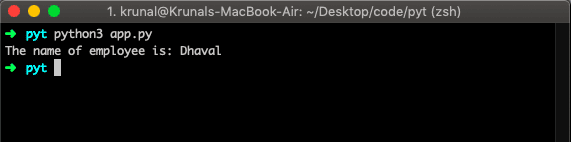## Encapsulation in Python

Using OOP in Python, we can restrict access to methods and variables. Encapsulation prevents data from direct modification.

In data encapsulation, we can not directly modify the instance attribute by calling an attribute on the object. It will make our application vulnerable to hackers. Instead, we only change the instance attribute values by calling the specific method. It could be setter or user-defined.

Let see the following example.

```# app.py

class Computer:

def __init__(self):
self.__maxprice = 1000

def sell(self):
print('Laptop Selling Price: {}'.format(self.__maxprice))

def setMaxPrice(self, price):
self.__maxprice = price

laptop = Computer()
laptop.sell()

laptop.__maxprice = 1100
laptop.sell()

laptop.setMaxPrice(1100)
laptop.sell()```

The output is below.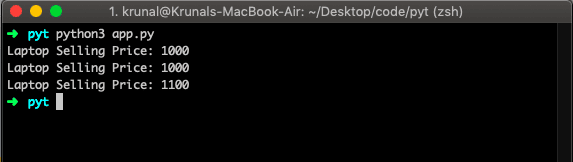In the above example, we have created an instance of Computer class and try to modify the instance variable’s value, but it still gives the value set inside the constructor.

We can only modify the instance attribute’s value by calling the setMaxPrice() method.

## Inheritance in Python

Inheritance is the way of creating a new class for using the data like attributes and methods of an existing class without modifying it. The newly formed class is the derived class or child class. Similarly, the existing class is the base class or parent class. See the following example.

```# app.py

class Bird:

def __init__(self):

def whoisThis(self):
print('This is hugsy')

def swim(self):

class Penguin(Bird):

def __init__(self):
super().__init__()

def whoisThis(self):
print('Penguin')

def run(self):
print('Hugsy runs faster')

hugsy = Penguin()
hugsy.whoisThis()
hugsy.swim()
hugsy.run()```

In the above example, the base class is Bird, and the derived class is Penguin. The derived class inherits the functions of the base or parent class. We can see this from the swim() method.

Again, the derived or child class modified the behavior of the parent class.

Also, we have to use the super() function before the __init__() method. This is because we want to use the content of__init__()  method from the parent class into the child class.

So, this is how inheritance work in Python.

Finally, the Python Object Oriented Programming Example is over.

#### 1 Comment

1.nayan

Hii,i am Nayan. python ke softwere me aap upar three dot kahase late ho.

This site uses Akismet to reduce spam. Learn how your comment data is processed.